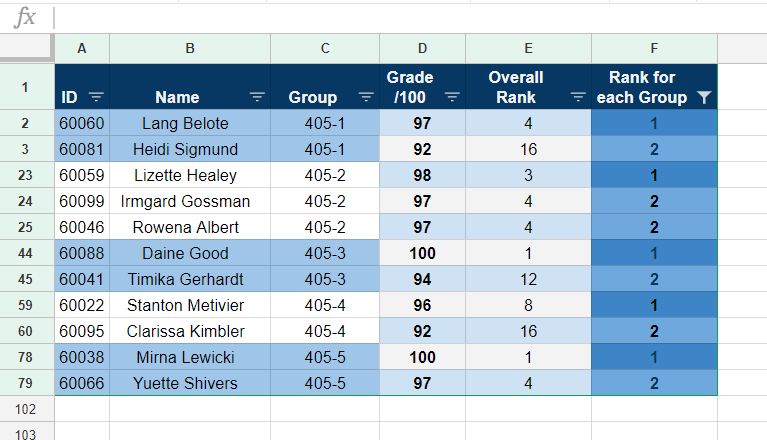# Google Sheets – Get the Top 2 Grades for Each Group in a Spreadsheet using RANK and FILTERThis week I was asked to provide a list of the top two student grades in each of the 100 classes in our university program.

Normally, this would be a pretty easy task if there were only a half dozen or so classes. Simply sort by group (class) and then Grade. Finally, copy and paste the top 2 ranked students of each group in a new sheet – print and hand to the boss.

However, with 100 classes this was going to turn into a time-consuming chore and one that could be prone to errors.

To solve this problem, I took advantage of Google Sheets RANK.EQ and FILTER formulas. Let’s take a peek at the formula now and go through the steps in detail later.

Let’s move on to an example.

### The Data

My example data here has 5 groups (or classes) with the student’s name, their id and grade.

### 1. Sort the Data

First, let’s tidy things up by sorting the data. We’ll want to sort the data by Group (ascending order) and then Grade (in descending order).

### 2. Add Overall Rank for All Students (optional)

This is an optional step, just to get us familiar with the RANK.EQ formula in Google Sheets. In Column E we are going to add an overall ranking for all students on the course. To do this, we use RANK or RANK.EQ. They are essentially the same.

RANK.EQ displays a specific rank of a value compared to a set of data. RANK.EQ takes 3 arguments.

1. Value: this is the value you want to compare against all other numbers in your set.
2. Dataset: This is the total range of values to use to make your comparison.
3. Ascending or Descending: You can leave this argument blank and it will automatically be ascending in rank order alternatively making this value FALSE will rank thinks in a descending order i.e. biggest number first.

Look at the first row of our example. We’ll add the first rank formula in Cell “E2“.

In cell E2 we want the rank of the grade in cell D2 against all the grades in Column D. We can see in the image above that student Lang Belote is ranking 4th for the course overall.

Let’s add this formula to all the rows in Column E.

Woah!!!! Easy There!!! There is more than one 4th ranked item!!!

Yep. Correct. If there are items in a dataset that have the same value they will be ranked the same. Then the next rank after those duplicates with be the previous rank plus the number of times it is repeated. A simple example would look like this.

### 3. Rank Grades for Each Group

Now that we have a handle on RANK, let’s add the FILTER formula so that we can simply rank grades within each group.

Here we select the cell that the grade we want to rank is in. Then in the dataset argument, we add the FILTER. FILTER takes:

1. Range: The range you want to use. In our case, this is column D, the Grade Column.
2. Filter Condition: This takes the range you want to filter by and the rule you want to use to filter. In our case, we want to limit our ranking to only those students in the same section. So we select Column C, the Group column and make sure it only filters out those grades who belong to the row we are working on (C2).

Let’s add this formula to the rest of the rows in Column F. I’ve added some Conditional Formatting to the first and second rank so you can see them more clearly.

### 4. Filter Only the Top 2 Grades for Each Group.

Finally, we want to only see the top 2 grades by each group. To do this:

1. Select all the data (ctrl+A)
2. Click on the Filter Button
3. Select the Filter drop-down in column F, Rank for Each Group.
4. Clear the selection.
5. Select only 1 and 2
6. Done.

That’s it! Done! You now have a list of the top 2 students from each group.

The good news is that you can now simply change the filter of Column F to view the lowest-performing students or failing students. Alternatively, you can now list the top 5 students for each class. Give it a try!

Give it a try.

Did you enjoy the tutorial? Want a solid step-by-step course to become a pro at Google Sheets? Check out my course, Google Sheets: Learn the Essentials with Three Detailed Projects. Sign up today.